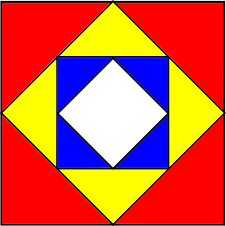# Exam-Style Questions.

## Problems adapted from questions set for previous Mathematics exams.

### 1.

IGCSE ExtendedThe diagrams above show a growing fractal of triangles. The sides of the largest equilateral triangle in each diagram are of length 1 metre.

In the second diagram there are four triangles each with sides of length $$\frac{1}{2}$$ metre.

In the third diagram there are 16 triangles each with sides of length $$\frac{1}{4}$$ metre.

(a) Complete this table for more diagrams.

 Diagram 1 Diagram 2 Diagram 3 Diagram 4 Diagram 5 Diagram 6 Diagram $$n$$ Length of Side 1 $$\frac{1}{2}$$ $$\frac{1}{4}$$ Power of 2 20 2-1 2-2

(b) Complete this table for the number of the smallest triangles in diagrams 4, 5 and 6.

 Diagram 1 Diagram 2 Diagram 3 Diagram 4 Diagram 5 Diagram 6 Diagram $$n$$ Number of smallest triangles 1 4 16 Power of 2 20 22 24

(c) Calculate the number of the smallest triangles in the diagram where the smallest triangles have sides of length $$\frac{1}{256}$$ metre.

### 2.

IB Standard

A square is drawn with sides of length 32 cm. The midpoints of the sides of this square are joined to form a new square and four red triangles. The process is repeated to produce yellow triangles and then again to produce blue triangles.The length of the equal sides of the red triangles are denoted by $$x_1$$ and their areas are each $$A_1$$.
The length of the equal sides of the yellow triangles are denoted by $$x_2$$ and their areas are each $$A_2$$.
The length of the equal sides of the blue triangles are denoted by $$x_3$$ and their areas are each $$A_3$$.

(a) The following table gives the values of $$x_n$$ and $$A_n$$, for $$1\le n\le3$$. Copy and complete the table.

 $$n$$ 1 2 3 $$x_n$$ 16 $$A_n$$ 128

(b) The process of drawing smaller and smaller squares inside each new square is repeated. Find $$A_7$$

(c) Consider an initial square of side length $$k$$ cm. The process described above is repeated indefinitely. The total area of one of each colour triangles is $$k$$ cm2. Find the value of $$k$$.

### 3.

IB Standard

The first term of an infinite geometric sequence is 10. The sum of the infinite sequence is 500.

(a) Find the common ratio.

(b) Find the sum of the first 9 terms.

(c) Find the least value of n for which Sn > 250.

### 4.

A-Level

In a geometric series the common ratio is $$r$$ and sum to $$n$$ terms is $$S_n$$.

Given that $$S_4 = \frac{8}{9} S_{\infty}$$ and $$r = \pm \frac{1}{\sqrt{k}}$$ find the value of $$k$$.

### 5.

IB Studies

Chris checks his Twitter account and notices that he received a tweet at 8:00am. At 8:05am he forwards the tweet to four people. Five minutes later, those four people each forward the tweet to four new people. Assume this pattern continues and each time the tweet is sent to people who have not received it before.

The number of new people who receive the tweet forms a geometric sequence:

$$1 , 4 , …$$

(a) Write down the next two terms of this geometric sequence.

(b) Write down the common ratio of this geometric sequence.

(c) Calculate the number of people who will receive the tweet at 8:40am.

(d) Calculate the total number of people who will have received the tweet by 8:40am.

(e) Calculate the exact time at which a total of 5 592 405 people will have received the tweet.

If you would like space on the right of the question to write out the solution try this Thinning Feature. It will collapse the text into the left half of your screen but large diagrams will remain unchanged.

The exam-style questions appearing on this site are based on those set in previous examinations (or sample assessment papers for future examinations) by the major examination boards. The wording, diagrams and figures used in these questions have been changed from the originals so that students can have fresh, relevant problem solving practice even if they have previously worked through the related exam paper.

The solutions to the questions on this website are only available to those who have a Transum Subscription.

Exam-Style Questions Main Page

Search for exam-style questions containing a particular word or phrase:

To search the entire Transum website use the search box in the grey area below.